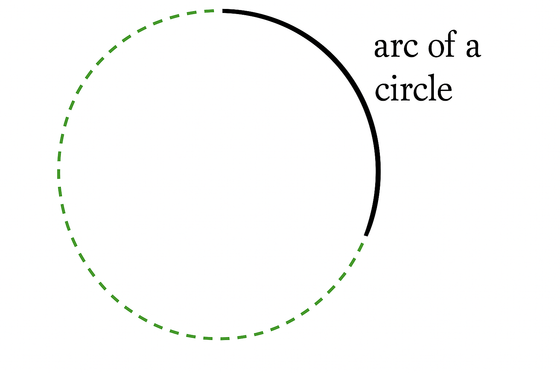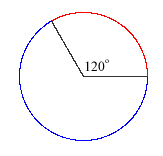index: click on a letter A B C D E F G H I J K L M N O P Q R S T U V W X Y Z A to Z index index: subject areas numbers & symbols sets, logic, proofs geometry algebra trigonometry advanced algebra & pre-calculus calculus advanced topics probability & statistics real world applications multimedia entrieswww.mathwords.com about mathwords website feedback

Arc of a Circle

A connected section of the circumference of a circle.Arcs are measured in two ways: as the measure of the central angle, or as the length of the arc itself.

 Measurement by central angle (degrees)The red arc (minor arc) measures 120°. The blue arc (major arc) measures 240°. Measurement by arc length (radians)Formula: s = rθ s = arc length r = radius of the circle θ = measure of the central angle in radians Red arc:  r = 2 and θ = 2π/3, so s = 4π/3 Blue arc:  r = 2 and θ = 4π/3, so s = 8π/3